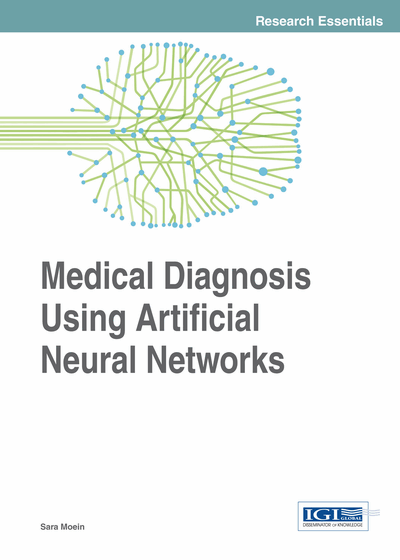# Training Algorithms

Copyright: © 2014 |Pages: 17
DOI: 10.4018/978-1-4666-6146-2.ch006

## Abstract

The process of assigning the weight to each connection is called training. A network can be subject to supervised or unsupervised training. In this chapter, supervised and unsupervised learning are explained and then various training algorithms such as multilayer perceptron (MLP) and Back Propagation (BP) as supervised training algorithms are introduced. The unsupervised training algorithm, namely Kohonen's self-organizing map (SOM), is introduced as one of most popular neural network models. SOMs convert high-dimensional, non-linear statistical relationships into simple geometric relationships in an n-dimensional array.
Chapter Preview
Top

## 2 Supervised Learning

Synapses interconnect the individual neurons through to make a network. As information is processed, the connections allow the neurons to signal each other. One weight is assigned to each connection and connections are not equal. The connection weight is zero, if there is no connection between two neurons. These weights are what determine the output of the neural network. Therefore, it can be said that the connection weights form the memory of the neural network.

The process of assigning the weight to each connection is called training. The beginning of the most training algorithms is by assigning random numbers to the weight matrix. Then the validity of the neural network is examined. Next, based on the valid performance of the network the weights are adjusted. This process is repeated until the validation error is within an acceptable limit. There are many ways to train neural networks. Neural network training methods generally fall into the categories of supervised, unsupervised and various hybrid approaches.

Supervised training is accomplished by giving the neural network a set of sample data along with the anticipated outputs from each of these samples. Supervised training is the most common form of neural network training. As supervised training proceeds, the neural network is taken through several iterations, or epochs, until the actual output of the neural network matches the anticipated output, with a reasonably small error. Each epoch is one pass through the training samples.

Supervised learning or Associative learning in which the network is trained by providing it with input and matching output patterns. These input-output pairs can be provided by an external teacher, or by the system, which contains the neural network (self-supervised).

It is very important to understand how to properly train a neural network. This book explores several methods of neural network training, including Back Propagation, Particle Swarm Optimization (PSO), and genetic algorithms. Once the neural network is trained, it must be validated to see if it is ready for use.

## Complete Chapter List

Search this Book:
Reset